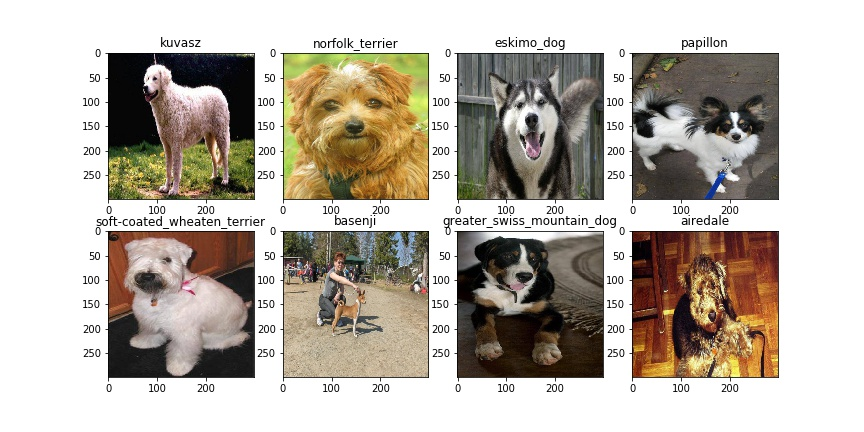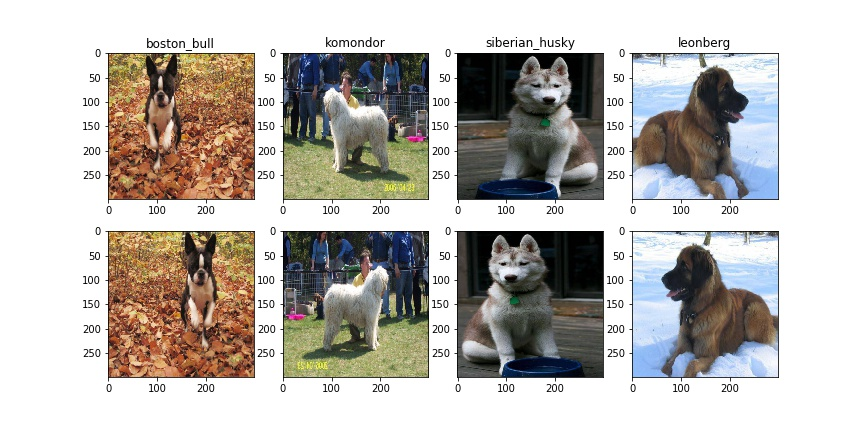# Transfer learning for image classification with Keras

Transfer learning from pretrained models can be fast in use and easy to implement, but some technical skills are necessary in order to avoid implementation errors. Here we describe a fast and easy to understand procedure using data from Kaggle’s Dog Breed Identification competition as an example. The same principles can be applied on virtually any image classification problem.

Since the code for this post is arguably lengthy, we focus only on the methodology here, providing the complete Jupyter notebook at Github. Our point of departure is a relevant kernel at Kaggle, which we improve, expand, and build upon.

From the three major transfer learning scenarios used in practice, here we are going to employ the first one, i.e. pretrained convolutional neural networks as feature extractors.Figure 1. Random sample of 8 dogs from Kaggle Dog Breed Identification competition

#### Software and libraries Used

For this task, we use Python 3, but Python 2 should work as well.

Our neural network library is Keras with Tensorflow backend. The pretrained models used here are Xception and InceptionV3 (the Xception model is only available for the Tensorflow backend, so using Theano or CNTK backend won’t work). We choose to use these state of the art models because of their very high accuracy scores.

We also use OpenCV (cv2 Python library) for image reading, Numpy for data preparation & preprocessing, and Pandas for reading & writing CSV files.

#### Preprocessing

We use OpenCV for image reading and resizing to 299×299, as this is the size that Inception and Xception models were initially trained. Since OpenCV reads images by default with BGR channel ordering while both our models require images with RGB channel ordering, we have to convert BGR to RGB. After that, we further preprocess the images using the utility function `preprocess_input` provided by Keras.

We also take advantage of the very convenient Numpy function flip, in order to quickly produce horizontal image flippings, as shown in Fig. 2 below.Figure 2. 4 random breed images (top) and their horizontal flip (bottom)

#### Methodology

We depart from a straightforward confrontation of the problem in three ways, i.e. regarding the use of extracted features,  cross-validation, and data augmentation; in particular:

• We concatenate features extracted using both of the state of the art models mentioned above, i.e. Xception and InceptionV3.
• We perform 5-fold cross-validation with an early stopping rule depending on the validation error, in order to avoid overfitting.
• During our 5-fold cross-validation, we actually use each of the 5 intermediate models produced in order to generate predictions for the test set, thus ending up with 5 different models whose outputs we are going to average.
• We repeat the above procedure, this time with the flipped images, thus ending up with test set predictions from 5 more models (i.e. 10 models in total).
• Our final submission is the averaged output from these 10 models.

#### Implementation

• Load train images in 2 numpy arrays `x_train`, `y_train`, as we only have 10,222 images and not too much RAM is needed. Also, in this way we avoid using a custom batch generator.
• Use the function `get_features` (see code snippet in the next section) to extract 2048 features from the last layer of each model. Concatenate features and create a 10,222×4096 numpy array. Create a second 10,222×4096 numpy array by extracting features from horizontally flipped images.
• To clear some RAM, delete train images as they are no longer needed and keep only extracted features.
• Do the same for test data (10,357 images).
• Do a 5-fold cross validation prediction with both the original and the flipped image features; this way we use all (100%) of training data, and also we average test predictions from 10 different models (1 model per fold for both original and flipped image). Fine tune each model using as input 4096 extracted features of 10,222 images, a dropout layer, and a dense layer of 120 classes for a maximum of 50 epochs, depending on early stopping.
• Create submission file.

#### Some details

We provide the full Jupyter notebook for this post at Github. Here we will only focus on the feature extraction and concatenation part.

Here is the, arguably pretty straightforward, code for our `get_features` function:

```
def get_features(MODEL, data=x_train):
#Extract Features Function from GlobalAveragePooling2D layer
pretrained_model = MODEL(include_top=False, input_shape=(width, width, 3), weights='imagenet', pooling='avg')
inputs = Input((width, width, 3))
x = Lambda(preprocess_input, name='preprocessing')(inputs)
outputs = pretrained_model(x)
model = Model(inputs, outputs)
features = model.predict(data, batch_size=64, verbose=1)
return features
```

Having defined `get_features`, extracting the features from each pretrained network and concatenating them are just one-liner operations; here is the code for the training set:

```
# Extract features from the original images:
x_xception = get_features(Xception, x_train)
x_inception = get_features(InceptionV3, x_train)
features_train1 = np.concatenate([x_xception, x_inception], axis=-1)

# Extract features from flipped images:
x_xception = get_features(Xception, np.flip(x_train,axis=2))
x_inception = get_features(InceptionV3, np.flip(x_train,axis=2))
features_train2 = np.concatenate([x_xception, x_inception], axis=-1)
```

#### Score propagation

With only one fold, training on 80% of the data our Multi Class Log Loss is lower than 0.27. Using 5 fold cv, the loss drops down to 0.24+. Adding the horizontal flips further improves our score to 0.23+.